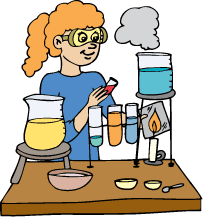## Monday, October 22, 2012

### Celebrate Mole Day in the ClassroomKnow the math behind chemistry? Then you’ve likely heard of Avagadro’s number (6.023 × 1023) that’s used as a basic unit of measure in chemistry. This number is more commonly referred to as a mole.

As you might guess, Mole Day is observed on October 23rd from 6:02 am to 6:02 pm, and can include anything mole related from eating molé sauce to playing a game of Whack-a-Mole. The punnier, the better.

In  Excel Math, we help students apply math to everyday life. For over 30 years, Excel Math has produced excellent results, including improved test scores and high student engagement with elementary mathematics—all while giving students a solid foundation of math skills. Take a quick tour of Excel Math here. Now fix yourself some mole sauce using either of the recipes below. Enjoy a snack break with some guaca-mole while you read more about moles, mole day and Excel Math.

Molé sauce (pronounced MO-lay) is a mexican tradition, and often includes chiles, oregano, cumin, garlic, chocolate and cinnamon, among other spices. With countless regional variations, this sauce can be green, yellow or red. It's usually served over smoked beef, chicken or enchiladas. Your class can try this easy recipe for Mole Sauce and then taste it as a dipping sauce for tortillas. Use the recipe as a math problem by having your students triple the recipe or divide it in half. Calculate how many tortillas you'll need if each student in the class gets a half. Then find out how many you would need for 3 classes with 25, 26 and 30 students in each class. If you're looking for a more authentic molé sauce recipe, try this one.

One teacher created this cute fabric mole pattern as a way for students to earn extra credit. You can download the PDF file here: Make-a-Mole-pattern:Fabric Mole Pattern Click here to download the pattern
Using the fabric of their choice, students can make a small stuffed toy mole to help them remember that a mole in chemistry is actually 6.023 × 1023. The photo below was taken after Ms. Kristy Gilmore's General Chemisty class at Hillcrest High School in Missouri made moles last year. Read more about their creations here.

To study mole-cules and density, use this activity from Excel Math Grade 6 to give your students a hands-on lesson. Bring two clear glass beakers labeled A and B, two ice cubes, 200 ml of water and 200 ml of isopropyl alcohol. Explain that density is a measure of the amount of molecules in a given object or volume. Draw two empty squares on the the board. Draw five large dots in one square and 10 large dots in the other. Ask the students if they can tell which box has a higher density. Help them understand that an object with a lot of molecules has a high density while an object with fewer molecules has a lower density.Ask the students what would happen if you dropped an ice cube into a glass of water. Would it float or sink? Explain that fluids like water also have densities. Ask them if density might play a role in this question. If so, how? (Ice has a lower density than water. Objects with lower densities than water float to the top.) Ask the students what would happen if you put the ice cube into another liquid that has a different density than water? Fill Beaker A with 200 ml of water and fill Beaker B will 200 ml of alcohol. Do not let the students know which liquid is in which beaker.
Hand out the lesson sheet below. Have the students write their observations about the two beakers on the lines provided. (Do not let the students smell the liquids.) Write a few of their observations on the board. Show them the two ice cubes. Explain that they are exactly alike. Ask them what they predict will happen when you drop the ice cubes in the beakers. Have the students write their predictions on the lines provided. Write a few of their observations on the board.

Drop the ice cubes in the beakers. Show the students how one ice cube floats to the top while the other sinks. Help the students understand that the liquids must be different. Based on your previous discussion, ask them which beaker contains water. Then ask them to explain the relationship between the densities of the ice cube and the liquid in Beaker B. Have them write their answer to question C on their papers. Then talk about the answer, explaining that the ice cube has a lower density than water but a higher density than alcohol.Excel Math Density Worksheet Click here to download a PDF file.